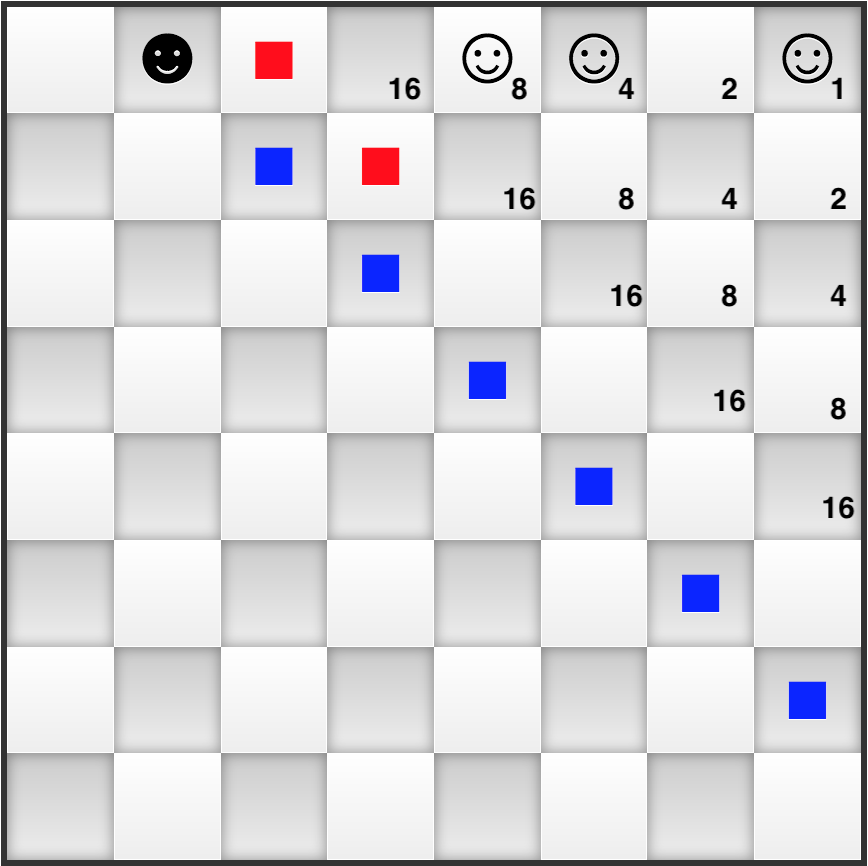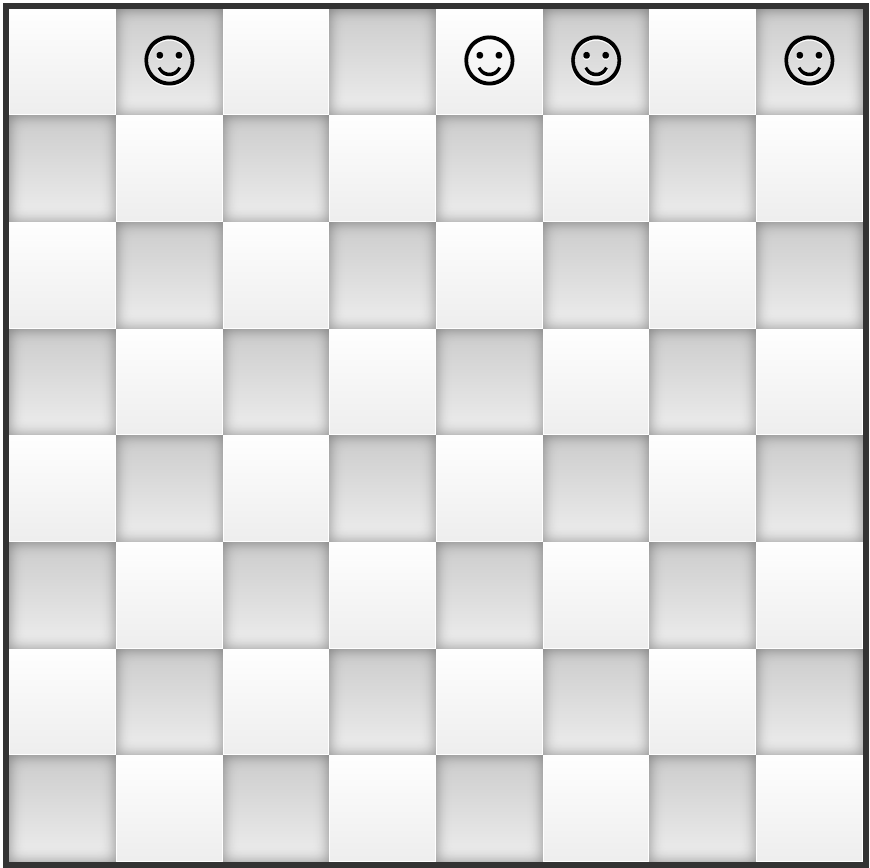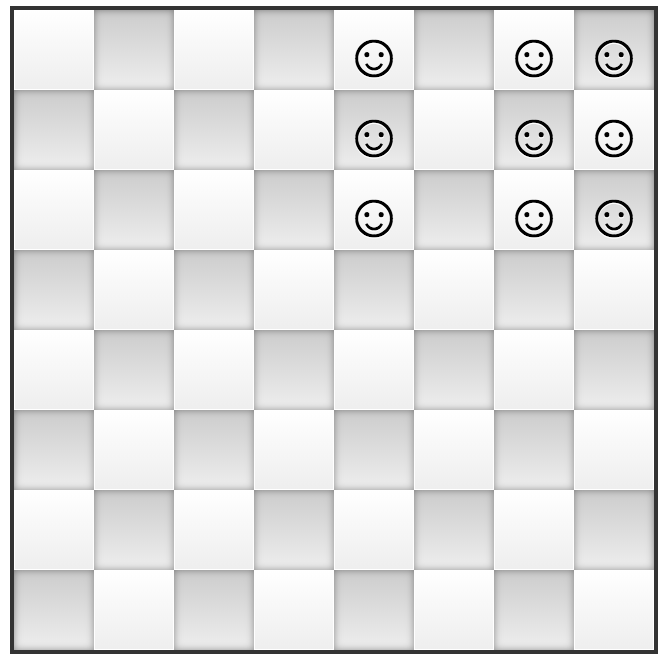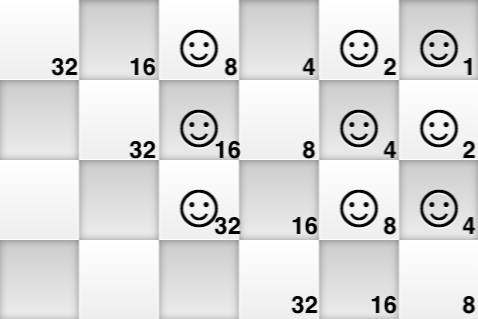# Why Is This Game Hard?

In the previous post we introduced the Integer Factorization game and also provided a JavaScript implementation so that you can actually play it online. We made the claim that this game is as hard as factorizing integers. In this post we'll show why this is the case and also show how to build hard to solve initial positions.

### Each position encodes a (move invariant) number

Recall that the goal of the game is to reach a final position from an initial one by moving tokens on the top-left bottom-right diagonals (the "blue" moves) or duplicating them on the next-higher diagonal (the "red" moves). For a given position let's assign numbers to all diagonals as shown in the following image:and then compute the "number" for this position by assigning to each token the value of its top-left bottom-right diagonal and then summing all token values. For example the above position's number is 1 + 4 + 8 + 64 = 77. It should be clear that moving a token on the same top-left bottom-right diagonal (the "blue" move) will not change this value. The "red" move maintains this number, too. For example, replacing the token on the diagonal "8" with two new tokens on the "4" diagonal will keep the invariant: 1 + 4 + 4 + 4 + 64 = 77.

### The connection with the binary multiplication

In the following image you could actually see an initial and a final position that have the same invariant number:
1 + 4 + 8 + 64 = 1 + 2 * 2 + 2 * 4 + 2 * 8 + 16 + 32 = 77.⇒⇒⇒You might also observe that 77 = 11 * 7 and this equality in binary is 1001101 = 1011 * 111. If we shift the rows to left until diagonals become columns, as shown in the following imageshould be relatively easy to see that the tokens in the final position are actually depicting the  1011 * 111  binary multiplication method taught in school:

``````   1011
x 111
-----
1011
1011
1011
-------
1001101
``````

### The conclusion

Based on the above observations we figure out that solving a game position is equivalent to factorizing the corresponding position's number. What we actually do when playing the game is the reverse of the binary multiplication method taught in school: we start with a position encoding a number in binary, for example 1001101, and we try to move or duplicate the tokens to arrive to a position representing some binary multiplication, for example 1011 * 111.

### Hard initial game positions

Obviously the game as implemented here is not that difficult to solve as we cannot have initial positions encoding numbers larger than 215 - 1. Solving positions on larger boards is a different problem though. At the time of writing there is a \$200,000 cash prize for factorizing this RSA-2048 number:

``````
RSA-2048 = 2519590847565789349402718324004839857142928212620403202777713783604366202070
7595556264018525880784406918290641249515082189298559149176184502808489120072
8449926873928072877767359714183472702618963750149718246911650776133798590957
0009733045974880842840179742910064245869181719511874612151517265463228221686
9987549182422433637259085141865462043576798423387184774447920739934236584823
8242811981638150106748104516603773060562016196762561338441436038339044149526
3443219011465754445417842402092461651572335077870774981712577246796292638635
6373289912154831438167899885040445364023527381951378636564391212010397122822
120720357.
``````

We can encode this number as an initial game position by using a chess board of size 1025 and, based on the fact no one managed to factorize RSA-2048 yet, this game position should be hard to solve.
But why is integer factorization a hard problem? We will discuss this topic in our next post.